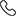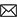Sales :9214233303 / Tech. Support Desk :9214233360/40 (9AM ~ 6PM)# Foundation Circle and heron''s formula, Constructions for class 9th by MC Sir

This course include deep conceptual details about circle,Discussion of Locus of point, Locus of circle, angle bisector, Derivation of equation of circle in cartesian form, proof of each theorem related to circle, condition of common points in circle such as 0 point common, 1 point common, and 2 point common, proof of theorem centroid divide the divide the median in 2 : 1, Conceptual discussion of each type of problem. Area of triangle by Heron's Formula, proof of heron's formula, Application of heron's formula.

• Rs.1,700 Rs.1,700
• Rs.2,700 Rs.2,700
• Rs.1,700 Rs.1,700
Subject Medium Target Language Mathematics English IX Class English + Hindi Topic Based 25 Videos 25 Videos PDF Files Full coverage of topic Circles and Heron's Formula 9th class Properly organized solutions in chapter-wise and page wise format help find your solution quickly Must for class 8th and 9th studying students who want to do well in NTSE, Olympiad, KVPY, IIT JEE, Medical and other engineering and competitive exams Ideal for class 9th students who are preparing for NTSE, Olympiad, KVPY, IIT JEE Exams. Essential for higher mathematics learning students. Essential for CBSE, NIOS & ICSE Exam preparations

### Other Courses by Manoj Chauhan SirTap a Star to Rate us:
close
close